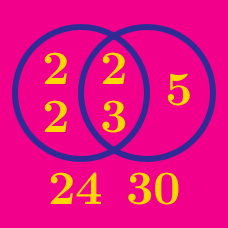Number Theory

# GCD and LCM - Problem solving

If the lowest common multiple of $4,6,8,9,$ and $x$ is $504,$ what is the third smallest possible integer $x?$

Note: Third smallest means the third value of $x$ if the possible values were listed from least to greatest.

What is the largest 3-digit number that is a multiple of 12 and a multiple of 21, but not a multiple of 22?

If the greatest common divisor of $266,308,392,$ and $x$ is $7,$ what is the sum of the smallest and largest possible 2-digit positive integers $x?$

$A$ and $B$ are positive integers such that $\begin{array}{c}&\gcd(A,B)= 9, &\mbox{lcm}(A,B) = 180, &A How many such $A$'s are there?

What is the lowest common multiple of the numbers 8, 9, 10, and 12?

×